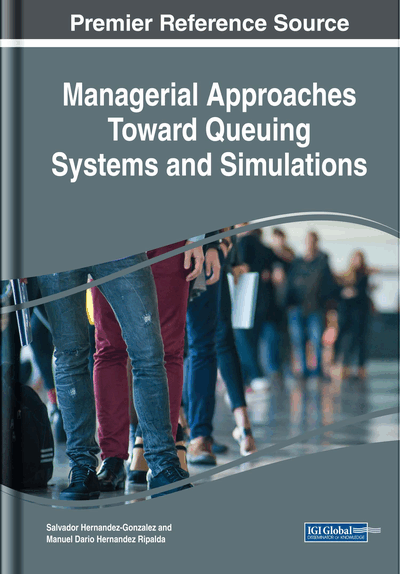# Review of Probability Distributions

DOI: 10.4018/978-1-5225-5264-2.ch002
Available
\$33.75
List Price: \$37.50
10% Discount:-\$3.75
TOTAL SAVINGS: \$3.75

## Abstract

In Chapter 2, probability distributions are presented; the distributions exposed are those with more relation to the analysis and study of waiting lines; discrete distributions: binomial, geometric, Poisson; continuous distributions: uniform, exponential, erlang, and normal. Confidence intervals are calculated for some of the parameters of the distributions. A brief example of the generation of pseudorandom exponential times using a spreadsheet is presented. The chapter closes with the goodness-of-fit tests of probability distributions, especially the Anderson-Darling test. The statistical language of programming R is used in the exercises performed. Several codes are proposed in R Language to perform calculations automatically.
Chapter Preview
Top

## Probability Distributions

When an experiment with random results is made. The set of possible events is called Sample Space. A function or mapping that identifies the events of the Sample Space with the set of real numbers is called Random Variable.

In general terms, a probability distribution could be called a rule for assigning probability values to random variables. Random variables can be classified in two types: discrete and continuous. Continuous variables are real numbers represented by X, while categorical variables are integers represented by N. Integers are a subset of real numbers.

• Cumulative Distribution Function: One particularly useful way of expressing probability distribution is by means of mathematical expressions: one is called probability density function, represented by f(x). The other type is called cumulative distribution function (CDF), represented by FX(x) or F(x), and assigns probabilities to intervals of the random variable.

A cumulative distribution function can be expressed as:

(1) where: F(x) is the cumulative distribution function; f(x) is the probability density function; X is a continuous random variable; “x” (lower case x) is a numeric value of interest. N is a discrete random variable and finally K is a continuous numeric value of interest.

Probability functions contain parameters that give every distribution different properties and behavior. These parameters are classify into three types: location parameters, scale parameters and shape parameters.

Every distribution has a different combination of parameters that, together with the functional form of the mathematical expression, gives different properties to every probability function.

Additional functions have been defined that help us to understand the behavior of a variable random. These functions are particular to each random variable. The ones most used in queues, are: expected value, variance and coefficient of variation:

It is often more practical to use the coefficient of variation squared, which in this case is:

(5)

If p=F(x), then q(p)= F-1(p)= x.

Given a sample of n randomly observed data, x1, x2, …, xn

## Complete Chapter List

Search this Book:
Reset